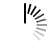# IntStream summaryStatistics() method in Java

The summaryStatistics() method in the IntStream class is used to return summary data about the elements of this stream. The syntax is as follows:

`IntSummaryStatistics summaryStatistics()`

Create an IntStream and add some elements:

`IntStream intStream = IntStream.of(30, 60, 90);`

Now, get the summary data about the above elements:

`IntSummaryStatistics details = intStream.summaryStatistics();`

The following is an example to implement IntStream summaryStatistics() method in Java:

## Example

Live Demo

```import java.util.stream.IntStream;
import java.util.IntSummaryStatistics;
public class Demo {
public static void main(String[] args) {
IntStream intStream = IntStream.of(30, 60, 90);
IntSummaryStatistics details = intStream.summaryStatistics();
System.out.println("Details = "+details);
}
}```

## Output

`Details = IntSummaryStatistics{count=3, sum=180, min=30, average=60.000000, max=90}`